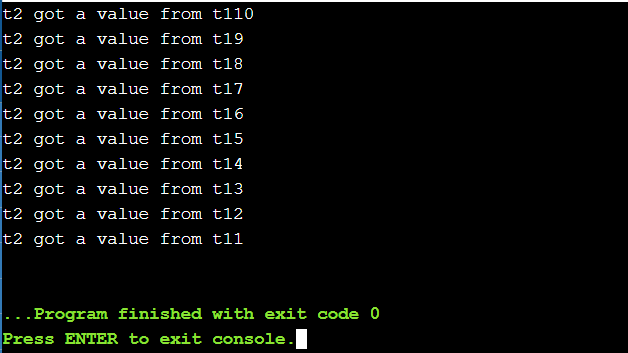# How to wake up a std::thread while it is sleeping?

• Difficulty Level : Hard
• Last Updated : 31 Mar, 2021

In this article, we will discuss how to wake up a std::thread while it is sleeping. It is known that a thread can’t be exited when it is sleeping. So it is woken up using a command as:

std::condition_variable

Want to learn from the best curated videos and practice problems, check out the C++ Foundation Course for Basic to Advanced C++ and C++ STL Course for foundation plus STL.  To complete your preparation from learning a language to DS Algo and many more,  please refer Complete Interview Preparation Course.

Below is the pseudo-code to implement the same:

## C++

 `// Custom Class``struct` `MyClass {`` ` `    ``// Constructor``    ``MyClass()``        ``: my_thread([``this``]() {``            ``this``->``thread``();``        ``})``    ``{``    ``}`` ` `    ``// Destructor``    ``~MyClass()``    ``{``        ``{``            ``std::lock_guard l(m_);``            ``stop_ = ``true``;``        ``}``        ``c_.notify_one();``        ``my_thread.join();``    ``}`` ` `    ``// Function that implements the``    ``// thread``    ``void` `thread``()``    ``{``        ``while` `(``this``->wait_for(std::chrono::minutes(2)))``            ``SendStatusInfo(some_info);``    ``}`` ` `    ``// Function to returns false when``    ``// the thread is stopped``    ``template` `<``class` `Duration>``    ``bool` `wait_for(Duration duration)``    ``{``        ``std::unique_lock l(m_);``        ``return` `!c_.wait_for(l, duration, [``this``]() {``            ``return` `stop_;``        ``});``    ``}`` ` `    ``// Conditions Variable``    ``std::condition_variable c_;``    ``std::mutex m_;``    ``bool` `stop_ = ``false``;``    ``std::``thread` `my_thread;``};`

Below is another example to illustrate the same:

std::promise/std::future

The above command can be used as a simpler alternative to the former method. In this case, a Future is not susceptible to false wakes and doesn’t require a mutex for synchronization. Below is the pseudo-code to implement the same:

## C++

 `// Promise Condition``std::promise<``void``> pr;`` ` `// Start the thread``std::``thread` `thr{``        ``[fut = pr.get_future()]{`` ` `            ``// Iterate until the condition``            ``// break``            ``while` `(``true``){``                ``if` `(fut.wait_for(std::chrono::minutes(2))``                    ``!= std::future_status::timeout) ``return``;``}``}``}``;`` ` `// When ready to stop``pr.set_value();`` ` `// Join the thread``thr.join();`

Below is the program to illustrate the above concepts:

## C++

 `// C++ program to illustrate the waking``// of the thread while it is sleeping``#include ``using` `namespace` `std;`` ` `std::deque<``int``> q;``std::mutex mu;``std::condition_variable cond;`` ` `// Function to create the thread 1``void` `function_1()``{``    ``// Initialize a counter variable``    ``int` `count = 10;`` ` `    ``// Iterate until count is positive``    ``while` `(count > 0) {`` ` `        ``// Mutex``        ``std::unique_lock locker(mu);`` ` `        ``// Push the current count in``        ``// the dequeue``        ``q.push_front(count);``        ``locker.unlock();``        ``cond.notify_one();`` ` `        ``// If there is any waiting thread``        ``// then notify that thread``        ``std::this_thread::sleep_for(``            ``chrono::seconds(1));`` ` `        ``// Decrement the count``        ``count--;``    ``}``}`` ` `// Function to create the thread 2``void` `function_2()``{``    ``// Initialize a variable to get``    ``// the data from the deque``    ``int` `data = 0;`` ` `    ``while` `(data != 1) {`` ` `        ``std::unique_lock locker(mu);`` ` `        ``cond.wait(locker, []() {``            ``return` `!q.empty();``        ``});`` ` `        ``// False Waking of thread``        ``data = q.back();``        ``q.pop_back();``        ``locker.unlock();`` ` `        ``// Print the message``        ``cout << ``"t2 got a value from"``                ``" t1"``             ``<< data << ``'\n'``;``    ``}``}`` ` `// Driver Code``int` `main()``{``    ``// Create thread 1``    ``std::``thread` `t1(function_1);`` ` `    ``// Create thread 2``    ``std::``thread` `t2(function_2);`` ` `    ``// Join the threads``    ``t1.join();``    ``t2.join();`` ` `    ``return` `0;``}`

Output:My Personal Notes arrow_drop_up